# Multi-Digit Multiplication

## Objective

Multiply four-digit numbers by one-digit numbers.

## Common Core Standards

### Core Standards

?

• 4.NBT.B.5 — Multiply a whole number of up to four digits by a one-digit whole number, and multiply two two-digit numbers, using strategies based on place value and the properties of operations. Illustrate and explain the calculation by using equations, rectangular arrays, and/or area models.

?

• 3.NBT.A.3

• 4.NBT.A.1

• 4.NBT.B.4

• 3.OA.B.5

• 3.OA.C.7

## Criteria for Success

?

1. Multiply a four-digit whole number by a one-digit whole number using area models and the partial products algorithm.
2. Understand that partial products can be written in increasing or decreasing order when recording them vertically.
3. Estimate products by rounding factors to the largest place value.
4. Solve one-step word problems involving multiplication of four-digit by one-digit numbers (on the Problem Set and Homework) (MP.4).

## Tips for Teachers

?

#### Remote Learning Guidance

If you need to adapt or shorten this lesson for remote learning, we suggest prioritizing Anchor Task 1 (benefits from worked example). Find more guidance on adapting our math curriculum for remote learning here.

#### Fishtank Plus

• Problem Set
• Student Handout Editor
• Vocabulary Package

?

### Problem 1

A student solved 4 x 2,387 below: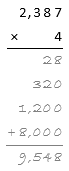a. How is this different from the way we recorded partial products previously?

b. Did this way of recording the partial products give the correct product? Use an area model and/or the way we recorded partial products yesterday to check.

### Problem 2

Estimate the product. Then use any method to solve.

a. 8 x 5,289

b. 3,067 x 6

## Problem Set & Homework

#### Discussion of Problem Set

• Can you represent #3 with an area model to solve even though it’s a problem involving arrays? Why or why not?
• Whenever there is a 0 in a factor, what does that mean about how many sections of an area model there will be? What about how many partial products there will be in the partial products algorithm?
• In #4, can you use the expression (6 x 5,000) + (6 x 400) + (6 x 70) + (6 x 9) to solve 6 x 5,479? How is this related to the partial products algorithm?
• I think the product in #2c is 1,490. What mistake do you think I made? What if I thought the answer was 149?
• Did you use any strategies from Lesson 11 to solve any problems on today’s Problem Set? For example, #2c or #5c?

?

### Problem 1

Which strategy for multiplying 5,712 and 4 should result in the correct product?

 A.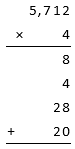B.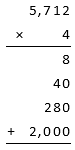C.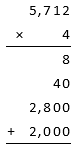D.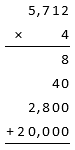#### References

Smarter Balanced Assessment Consortium: Item and Task Specifications Math Item Specifications - Claim 1, Grade 4 (G4_1E_NBT_Spec_v4)Task Model 2d, Example Stem

Math Item Specifications - Claim 1, Grade 4 (G4_1E_NBT_Spec_v4) from Development and Design: Item and Task Specifications made available by Smarter Balanced Assessment Consortium.  © The Regents of the University of California – Smarter Balanced Assessment Consortium. Accessed Dec. 11, 2018, 1:34 p.m..

Modified by The Match Foundation, Inc.

### Problem 2

Explain why the expression 8 x (3,000 + 600 + 5) can or cannot be used to find the value of 3,605 x 8.

#### References

PARCC Released Items Math Spring Operational 2016 Grade 4 Released ItemsQuestion #26, Part A

What is the value of 3,605 x 8?

#### References

PARCC Released Items Math Spring Operational 2016 Grade 4 Released ItemsQuestion #26, Part C

### Problem 3

A pencil is 7 inches long. The Empire State Building is 2,492 times as tall as a pencil. How tall, in inches, is the Empire State Building?

### Mastery Response

?